Click to Chat

1800-1023-196

+91-120-4616500

CART 0

• 0

MY CART (5)

Use Coupon: CART20 and get 20% off on all online Study Material

ITEM
DETAILS
MRP
DISCOUNT
FINAL PRICE
Total Price: Rs.

There are no items in this cart.
Continue Shopping• Complete JEE Main/Advanced Course and Test Series
• OFFERED PRICE: Rs. 15,900
• View Details

Structure of Atom

Subatomic Particles:

Name

Electron

Proton

Neutron

Symbol

e-

p

n

Approximate relative mass

1/1836

1

1

Mass in kg

9.109×10–31

1.673×10–27

1.675×10–27

Mass in amu

5.485×10–4

1.007

1.008

Charge (coulomb)

1.602×10–19

1.602×10–19

0

Actual Charge (e.s.u)

4.8 × 10–10

4.8 × 10–10

0

Atomic Models:

Thomson’s Atomic Model (Plum – pudding model):-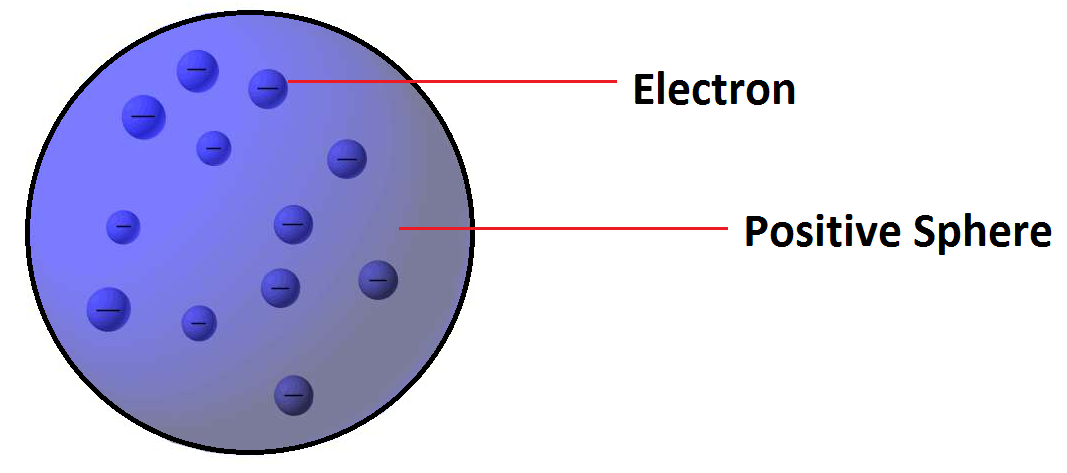Postulate: -Atom is a sphere of positive charge in which number of electrons are embedded.

Limitations: - Could not satisfactorily explain the results of scattering experiment carried out by Rutherford.

Rutherford’s Model:

Postulates:-

Almost all the positive charge and mass of atom is present in its nucleus.

Electrons revolve around the nucleus in circular orbits.

There is strong electrostatic attraction between nucleus and electrons

Limitations: - Could not explain stability and electronic structure of atom.

Atomic Terms

Terms

Definition / Explanation

Atomic Number (Z)

Number of protons or electrons of neutral atom.

Mass Number (A)

Total number of protons and neutrons in an atom

Nucleons

Protons and neutrons are present in a nucleus. So, these fundamental particles are collectively known as nucleons

Isotopes

Atoms of the element with same atomic number but different mass number e.g. 1H1, 1H2, 1H3.

Isobars

Atoms having the same mass number but different atomic numbers, e.g. 15P32 and 16S32

Isotones

Atoms having the same number of neutrons but different number of protons or mass number, e.g. 6C14, 8O16, 7N15

Isoelectronic

Atoms, molecules or ions having same number of electrons e.g. N2,CO, CN–

Nuclear isomers

atoms with the same atomic number

and same mass number but with

Example of nuclear isomers is

Uranium –X (half life 1.4 min) and

Uranium –Z (half life 6.7 hours

Isosters

Molecules having  same number of atoms and

also same number of electrons are called

isosters. E.g., N2  and CO

Wave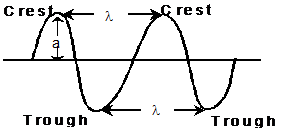Terms

Explanation

Wave length (λ)

Distance between two neighbouring troughs or crests.

Frequency (ν)

Number of times a wave passes through a given point in a medium in one second.

ν = c/λ

Velocity (c)

The distance travelled  by the wave in one second.

c = νλ

Wave number

Number of wavelengths per cm.

Amplitude (a)

Height of the crest or depth of the trough. Determines the intensity of the beam of light.

Electromagnetic Waves

Wave length (Å)

3×1014 to 3 ×107

Micro waves

3×109 to 3 ×106

Infrared (IR)

6×106 to 7600

Visible

7600 to 3800

Ultra violet (UV)

3800 to 150

X–rays

150 to 0.1

Gamma rays

0.1  to 0.01

Atomic spectrum of hydrogen atom: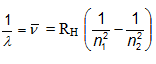Where, RH = Rydberg constant (108978 cm-1)

n1 and n2 have integral values as follows

n1

n2

Spectral Series

Spectral region

1

2,3,4…

Lyman

UV

2

3,4,5…

Balmer

Visible

3

4,5,6…

Pascher

IR

4

5,6,7…

Brackett

IR

5

6,7,8…

Pfund

IR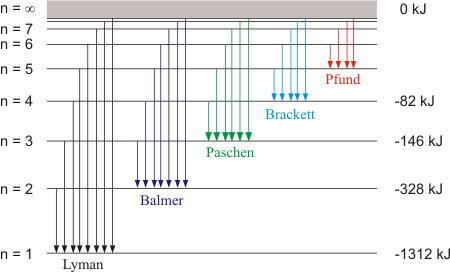Photoelectric effect: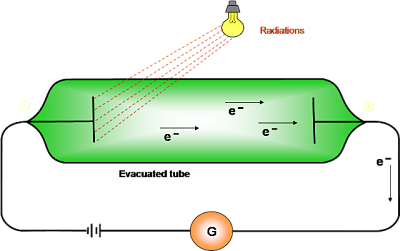Ejection of electrons takes place from the surface of metal when light of suitable frequency fall on it.

Minimum frequency required for ejection of electron is called threshold frequency (vo).

Energy of the ejected electrons is directly proportional to the frequency of radiation.

Number of electrons ejected per second depends on the intensity of radiation.

hv- hvo =1/2mev2

Planck’s quantum theory:

Substances radiate or absorb energy discontinuously in the form of energy packets

The smallest packet of energy is called quantum. In case of light the quantum is known as photon.

The energy of a quantum is directly proportional to the frequency of the radiation.

E = hv were v is the frequency of radiation and  h is Planck’s constant having the value 6.626 × 10–27 erg sec or 6.626 × 10–34 J sec.

A body can radiate or absorb energy in whole number multiples of quantum hn, 2hν,3hν………..nhν, where n is the positive integer.

Bohr’s atomic model:

Electrons revolve around the nucleus in circular orbits of fixed energy.

Electron revolve only in those orbits whose angular momentum (mvr) is an integral multiple of h/2Π.

Electron absorbs energy in the form of EMR, when it jumps from lower energy level (ground state) to higher energy level (excited state) and vice-versa.

Energy absorbed or released in an electron jump, (dE) is given by dE = E2 – E1 = hν

Energy of stationary state oh hydrogen atom (En) =  -RH (1/n2)

For an hydrogen like species i.e. He+, Li2+ with atomic number Z

Radius of nth orbit (rn ) = 52.9 × n2/z  pm

Energy of nth orbit (En) = -2.18×10-18(Z2/n2) = –13.6 ×(Z2/n2)  eV = 313.6 ×(Z2/n2) kcal /mole

Velocity of electron (v) = (2.18 ×108 ) z/n cms-1

Where n = 1,2,3,4…

Limitations of Bohr’s theory:

Failed to explain the spectra of atoms having more than one electron.

Failed to account for the splitting of spectral line source of a spectrum is placed in a strong magnetic or electric field.

Dual nature of particle and the uncertainty principle was ignored in the Bohr’s atomic model.

de- Broglie equation:

λ = h/mv = h/p

Heisenberg’s uncertainty principle:

It is impossible to determine simultaneously, the exact position and exact momentum of an electron.

Quantum Numbers:

Principal quantum number (n):

It tells the main shell in which the electron resides and the approximate distance of the electron from the nucleus.

Maximum number of electrons a shell can accommodate is 2n2.

Azimuthal or angular momentum quantum number (l):

It represents the number of subshells present in the main shell.

These subsidiary orbits within a shell will be denoted as s,p,d,f…

This tells the shape of the subshells.

For a given value of n, there are n possible values of l starting from 0 to (n-1)

Value of l

0

1

2

3

4

Notation of sub shell

s

p

d

f

g

The magnetic quantum number (m):

It determines the number of preferred orientations of the electron present in a subshell.

For a given value of l, there are (2l+1) possible values of m starting from –l to +l.

Value of l

0

1

2

3

Notation of sub shell

s

p

d

f

Values of m

0

-1,0,1

-2,-2,0,1,2

-3,-2,-1,0,1,2,3

The spin quantum number (s)

It determines the direction of spin of electron in an orbit.

There are only two possible values for spin quantum number i.e. -1/2 ,+1/2.

Rules for filling of electrons in various orbitals :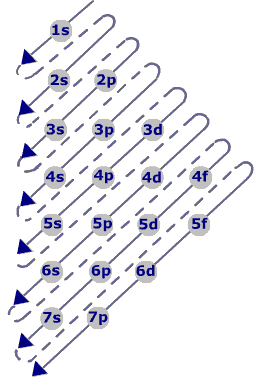Aufbau Principle:

Electrons are added one by one to the various orbitals in order of their increasing energy starting with the orbital of lowest energy.

In neutral isolated atom, the lower the value of (n+ l) for an orbital, lower is its energy

The increasing order of energy of various orbital is : 1s < 2s < 2p < 3s < 3p < 4s < 3d < 4p < 5s < 4d < 5p <  6s < 4f < 5d ..

Pauli’s Exclusion principle :-

An orbital can contain a maximum number of two electrons and these two electrons must be of opposite spin.

Hund’s rule of maximum multiplicity :-

Electron pairing in p, d and f orbital cannot occur until each orbital of a given subshell contains one electron each or is singly occupied”.

Exceptional Configurations

Stability of half filled and completely filled orbitals

Cu  has  29 electrons. Its expected electronic configuration is

1s2, 2s2, 2p6, 3s2, 3p6, 4s2, 3d9

But a shift of one electron from lower energy  4s orbital to higher energy 3d orbital will make the distribution of electron symmetrical and hence will impart more stability.

Thus the electronic configuration of Cu is

1s2, 2s2, 2p6, 3s2, 3p6, 4s1, 3d10

Fully filled and half filled orbitals are more stable### Course Features

• 728 Video Lectures
• Revision Notes
• Previous Year Papers
• Mind Map
• Study Planner
• NCERT Solutions
• Discussion Forum
• Test paper with Video Solution